High Level Approximation Quiz Part- 11Question 1.

What should come at the place of question mark (?) in the following question?A. 4
B. 6
C. 8
D. 7
E. 5
Ans. B.
Solution: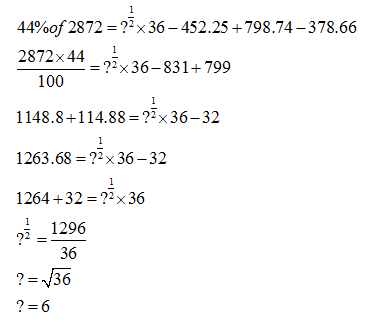Question 2.

What should come at the place of question mark (?) in the following question?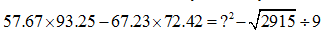A. 26
B. 36
C. 34
D. 24
E. 28
Ans. D.
Solution:Question 3.

What should come at the place of question mark (?) in the following question?A. 2
B. -1
C. -2
D. 3
E. -3
Ans. B.
Solution:Question 4.

What should come at the place of question mark (?) in the following question?A. 256
B. 16
C. 2744
D. 216
E. 4096
Ans. E.
Solution: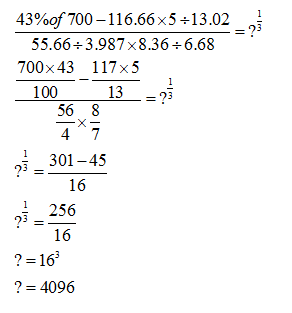Question 5.

What should come at the place of question mark (?) in the following question?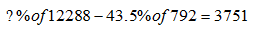A. 11.11%
B. 3.33%
C. 333.33%
D. 66.66%
E. 33.33%
Ans. E.
Solution:Question 6.

What approximate value should come in the place of question mark (?) in the following question: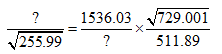A. 12
B. 24
C. 64
D. 36
E. 81
Ans. D.
Solution: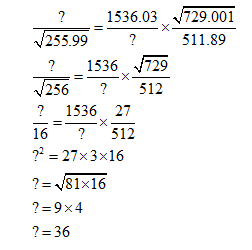Question 7.

What approximate value should come in the place of question mark (?) in the following question: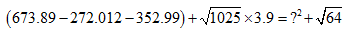A. 333
B. 17
C. 19
D. 13
E. 23
Ans. D.
Solution: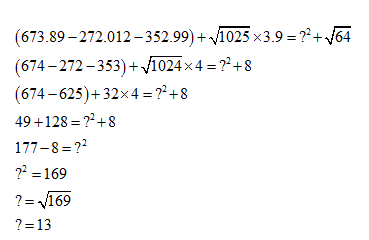Question 8.

What approximate value should come in the place of question mark (?) in the following question: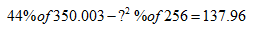A. 9
B. 3
C. 6
D. 5
E. 8
Ans. B.
Solution: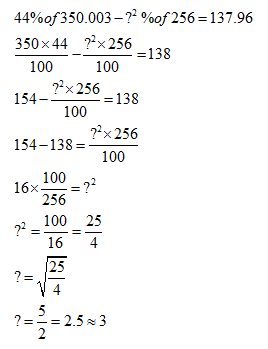Question 9.

What approximate value should come in the place of question mark (?) in the following question:A. 1156
B. 1024
C. 1728
D. 2401
E. 2744
Ans. E.
Solution:Question 10.

What approximate value should come in the place of question mark (?) in the following question:A. 169
B. 144
C. 196
D. 121
E. 81
Ans. D.
Solution:What's trending in BankExamsToday

Smart Prep Kit for Banking Exams by Ramandeep Singh - Download here

Join 40,000+ readers and get free notes in your email